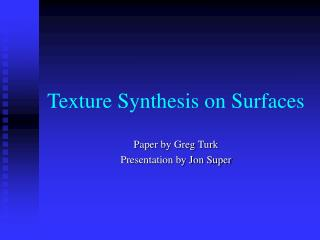DownloadDownload PresentationTexture Synthesis on Surfaces

# Texture Synthesis on Surfaces

Télécharger la présentation## Texture Synthesis on Surfaces

- - - - - - - - - - - - - - - - - - - - - - - - - - - E N D - - - - - - - - - - - - - - - - - - - - - - - - - - -
##### Presentation Transcript

1. Texture Synthesis on Surfaces Paper by Greg Turk Presentation by Jon Super

2. Goals of texture placement Take a 2D texture and wrap it onto a surface • Avoid noticeable seams between patches • Minimize stretching and distortion • Produce a natural-looking result

3. The next best thing Lapped Textures – Praun et al. – SIGGRAPH00 • Uses many overlapped texture patches • Aligns patches with underlying vector field • Generally minimizes distortion

4. The next best thing Lapped texture’s shortcomings • Low-frequency components • Boundary mismatch • Direction field singularities

5. Texture synthesis to the rescue!

6. Process • Create a mesh hierarchy over the model • Set up a vector field over the mesh • Calculate sweep distance for each vertex • Assign colors based on neighbors

7. Process • Create a mesh hierarchy over the model • Set up a vector field over the mesh • Calculate sweep distance for each vertex • Assign colors based on neighbors

8. Mesh Hierarchy Equivalent of a Gaussian pyramid to a surface • Multiple layers of different resolutions • Each layer has 4 times the number of vertices of the layer above it • Each layer contains every vertex of the layer above it

9. Point placement • n points randomly placed on the surface • Repulsion between the points spaces them evenly • These points are now fixed in place, representing level m of an m-level mesh • Repeated for each layer, adding 3 times more points each time (3n, 12n, etc)

10. Connectivity • Connectivity between vertices of each layer • Delaunay triangulation applied • Mesh is connected in a web of triangles

11. Mesh hierarchy operations • Interpolation • Low-pass filtering • Downsampling • Upsampling

12. Process • Create a mesh hierarchy over the model • Set up a vector field over the mesh • Calculate sweep distance for each vertex • Assign colors based on neighbors

13. Vector field • Provides order to the placement of the texture on the model • Each mesh vertex is given a vector • User initiates the process by defining a few key vectors (~12)

14. Vector field creation • All vertices not user-defined set to 0 • User-defined vectors are downsampled to the coarsest mesh • Low-pass filtering to fill out top mesh • Upsample for each layer to fill to bottom

15. Process • Create a mesh hierarchy over the model • Set up a vector field over the mesh • Calculate sweep distance for each vertex • Assign colors based on neighbors

16. Surface sweeping • Uses the directional flow of the vector field to assign ordering to the vertices • Ordering will allow a sort of raster scan over the 3D model • The color in the image cycles as the sweep distance increases

17. Sweep distance calculation • Select an anchor point to base the distance • Each vertex takes the value that is the weighted sum of what its neighbors think • Distance along the flow direction added to the neighbor’s sweep distance • Sweep distances will propagate out from the anchor until every vertex has one

18. Process • Create a mesh hierarchy over the model • Set up a vector field over the mesh • Calculate sweep distance for each vertex • Assign colors based on neighbors

19. Pixel Neighborhoods • Similar technique to 2D texture synthesis • Examine nearby colors and look for a similar pattern in the texture image • Different kinds of pixel neighborhoods

20. Mesh coordinate space • Pixel neighborhoods don’t translate directly to the unorganized vertices in a mesh • Vertex vector is down • Rotate 90 degrees CCW for right

21. Multi-level synthesis • Start at top, work down • Initialize the vertices of mesh layer k with color values from Gaussian pyramid layer k • Make a pass with the half square • Top layer should have a crude representation of the texture

22. Multi-level synthesis • Extrapolate and refine each lower level • Extrapolate initializes the colors using a large full square neighborhood of the level above • Refine improves on that by combining a large full square on the current level with a small full square on the level above

23. Algorithm

24. Results

25. Results

26. Results

27. Results

28. Results

29. Results